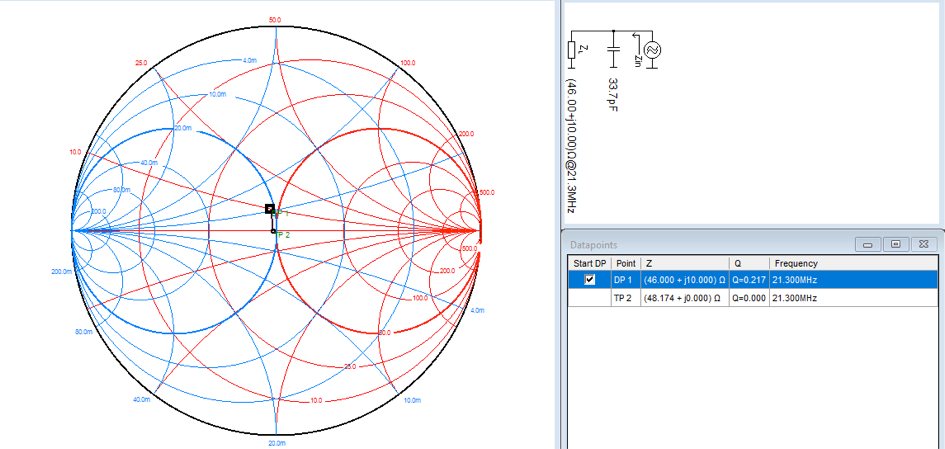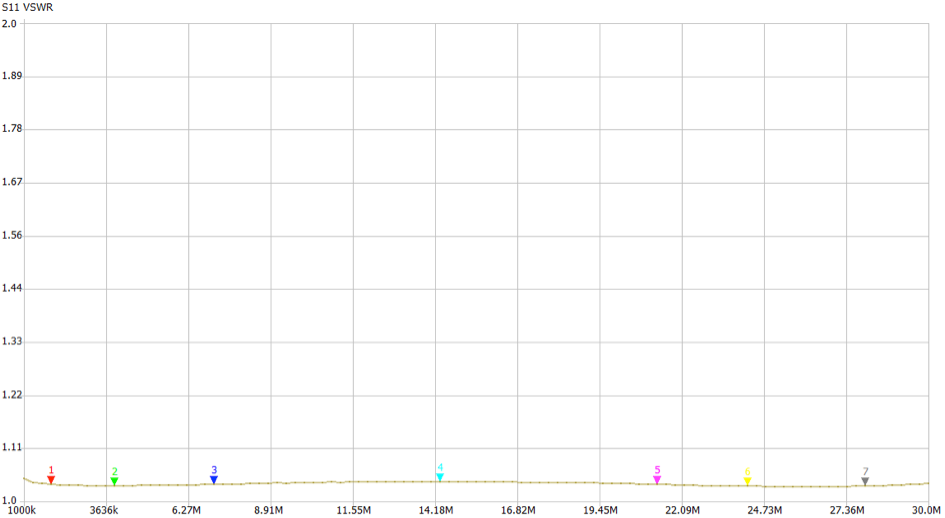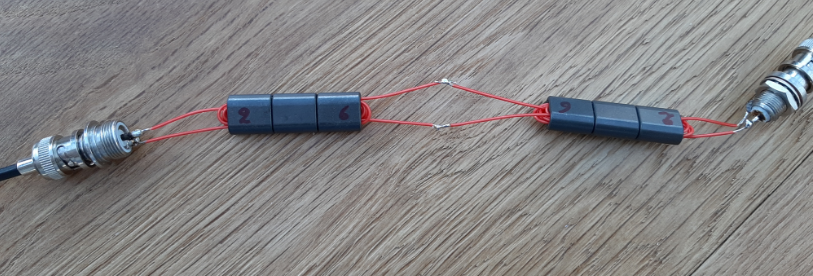Search
• xtgaby

# 9:1 transformer for K9AY antenna.

Updated: Mar 7

Picture Schematicsprimary 2 turns secondary 6 turns

Used material: 3 cores of 73 material Fair-Rite 2873000202Input = 2 turns / Output = 6 turns

2 square = 4 / 6 square = 36

36 / 4 = 9

When Input impedance = 50 Ohms,

Output impedance wil be 9 x 50 Ohm = 450 OhmsAt 21.3 MHz impedance is 46 ohms + j10 ohmsCompensation is 34 pF @ 21.3 MHzS21 Insertion loss for 2 devices:

The K9AY antenna is used at 160 meters and 80 meters.

The setup:Insertion loss at 160 meters =

0.33 dB / 2 = 0.17 dB

This means a loss of 4 %

Insertion loss at 80 meters =

0.35 dB / 2 = 0.18 dB

This means a loss of 4 %

S21 insertion loss for 2 devices: